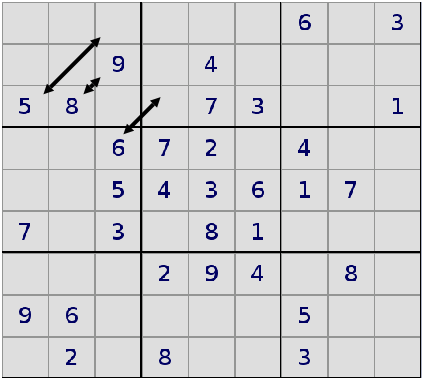# Similar Sudokus and Isomorphism

If you swap all 1s and 2s in a Sudoku puzzle, the Sudoku still follows the same logic.

Two Sudokus that follow the same logic are said to be similar or "isomorph"

For each Sudoku there are 2*9!*68 = 1218998108160 isomorph Sudokus.

## Transformations

You can apply the following transformations to a Sudoku without changing its logic.

### TranspositionTransposition is exchanging the x- and y-axis, that is exchanging the upper right number with the lower left ones. The logic of the Sudoku doesn't change if you transpose it.

The transformation of exchanging the lower left and upper right numbers is called transposition.

If you transpose a Sudoku (or a matrix) twice, you don't change anything.

Therefore there are two versions of each Sudoku: an original and a transposed version.

You should note that you have to exchange numbers with empty cells as well.

### Reordering Rows and Columns

If you change the sequence of rows or columns within a 3x3-Subgrid you don't affect a Sudoku's logic.When exchanging rows and cols within a 3x3-Subgrid the game's logic doesn't change.

Within a 3x3 sub grid the first column can be placed at three different positions, the second column still has two places to go, only one place is left for the third row.

Therefore you have 3*2*1 = 6 possibility of ordering the three cols.

You can exchange rows and columns in each 3 3x3 sub grids, so you have 66 overall possibilities.

### Reordering 3x9 / 9x3 sub gridsYou can reorder blocks of three adjacent rows or columns, as shown in the figure above.

Using the same considerations as above you find that there are 6*6 possibilities of doing this.

### Permutations

You can exchange numbers in a Sudoku without changing its logic, provided that you exchange all occurrences.

You can for example substitute each 8 by a 3, each 3 by a 5, and each 5 by a 3.

There are 9! = 9*8*7*6*5*4*3*2*1 = 362880 possibilities of permuting the 9 numbers.

You should note that in the previously discussed transformations all you had to exchange numbers with empty cells. When permuting the numbers, the empty cells stay unchanged.

With permuting you can get each Sudoku in a form so that the first 3x3 sub grid contain the numbers from 1 to 9 in ascending order:

 1 2 3 4 5 6 7 8 9

## Summary

There are 2 possibilities from transposition, 66 possibilities from reordering cols and rows, 6*6 possibilities from reordering blocks, and 9! permutations.

All together that are 2*68*9! = 1218998108160 related Sudokus.

top